# Circles Practice Questions: Level 02

1. The wheel of scooter has diameter 140 cm. How many revolutions per minute must the wheel make so that the speed of the scooter is kept at 132 km per hour?
A. 1100
B. 500
C. 250
D. 1000

2. In the figure AC and BC are radii of circles. The length of AB is 8. If AC = 4, what is BC? (BC is tangent to the circle with center A.)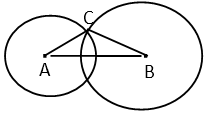A. 5
B. 5/2
C. 4√3
D. 3

3. Two circles with same center are drawn with O as the centre as shown is the figure given below. The ratio of the area of the annular ring bounded by these two circles and the quadrilateral EBCH is 3×:2. Find the ratio of the radius of the smaller circle to the radius of the larger circle.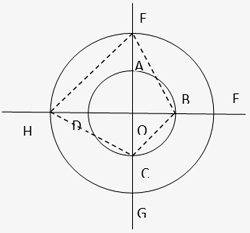A. 1:4
B. 2:7
C. 1:6
D. 1:7

4. A semicircle having centre at O and radius equal to 4 is drawn with PQ as the diameter as shown is the figure given below. OSRU is a rectangle such that the ratio of area of the semicircle to the area of the rectangle is 2π: 3 or cuts the semicircle at T. Find the length of line segment TQ.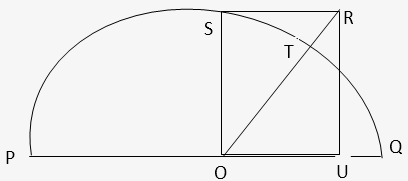A. (8/5)√5
B. (5/3)√5
C. (17/9)√5
D. (9/2)√5

5. A circle of radius 3 cm is drawn inscribed in a right angle triangle ABC, right angled at C. If AC is 10 Find the value of CB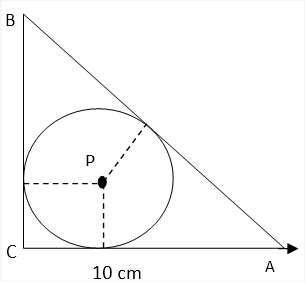A. 10.5cm
B. (20/7)√58 cm
C. 21/2 cm
D. None of these

1. In the circle shown below, AB is a diameter and CD is a chord. The lengths of AB and CD are integers, such that the length of CD is obtained by reversing the two digits of length of AB. If the length of OE is a rational number, find the length of OE.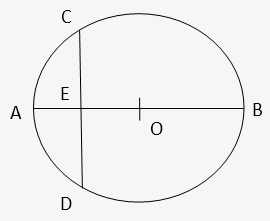A. 16
B. 16.5
C. 33
D. None of these

2. In the given figure, AB is the diameter of the circle with center O. If ∠BOD = 15° & ∠EOA = 85°, then find the value of ∠ECA.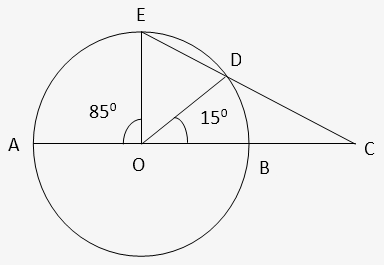A. 20°
B. 25°
C. 35°
D. Can’t be determined

3. The smallest possible circle, touching two opposite sides of a rectangle, is cut out from a rectangle of area 60 sq units. If the area of the circle is 1.5 times the uncut area left in the rectangle, find the diameter of the circle.
A. (6/√π) units
B. (9/√π) units
C. (12/√π) units
D. (15/√π) units

4. The line √3y = x meets the circle having the centre at origin O at point M(√3,1) . Referring to the diagram below, if PQ is a tangent to the circle at M, find the length of PQ.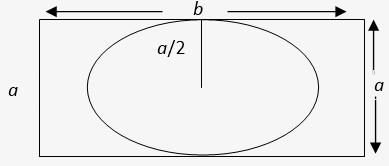A. (5/2)√3
B. 3√3
C. 2√3
D. 8/√3

5. In the figure O and O’ are the centers of the bigger and smaller circles respectively. The smaller circle touches the square ABCD at the midpoint of side AD. The radius of the bigger circle is 30 cm and the side of the square ABCD is 36 cm. Find the radius of the smaller circle.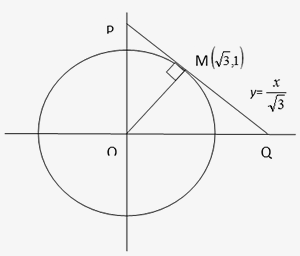A. 9.25 cm
B. 9 cm
C. 9.45 cm
D. 10 cm